Dapta

# Dynamic aeroelastic flutter and divergence analysis with Python and Calculix

This is a continuation of our parametric composite wing tutorial. This time, we add a dynamic aeroelastic stability analysis to our code, so that we can calculate critical flutter and divergence flight velocities. We start by extracting mass and stiffness information from our CalculiX model and then solve a complex eigenvalue problem with the help of the Scipy sparse matrix library.

The example source code can be found on github (v0.0.5.fix2) and there is also a youtube video run-through of the code updates, that includes a demo of the aeroelastic analysis implementation and some sample results.

#### What is flutter and why does it matter?

Flutter is a type of self-excited aeroelastic system vibration that can lead to catastrophic failures. Famously, the collapse of the Tacoma Narrows bridge in 1940 was due to flutter. Flutter or limit cycle oscillations are not actually an uncommon phenomenon (here an entertaining flutter compilation). However, a lot of work goes into designing it out of an aircraft’s certification flight envelope as much as possible and demonstrating that it doesn’t occur via testing and simulation.

Divergence is another type of instability that can happen when there is a quasi-static positive feedback loop between the deflections of the wing and the lift forces on it. Divergence is particularly a problem for aircraft with forward-swept wings, such as the X-29 (divergence explained on the X29).

#### Example part 6: Running the aeroelastic analysis

The video below covers the solution of our dynamic aeroelastic problem and how this was implemented in the parametric wing model code. We also execute the analysis and plot some of the modal histories that allow us to graphically identify flutter and divergence velocities.

#### Calculating the aerodynamic forces on the wing

In our example video above we introduce the basic aeroelastic equation that can be used to calculate the flutter and divergence velocities. But where exactly do we get our aero stiffness (C) and damping (B) matrices from that appear in that equation?

In the example we approximate the dynamic forces using a modified strip theory: we simply divide the wing along its span into a number of equally wide strips and then we independently calculate the lift force and moment on each of these strips as a function of the local strip deflections and velocities. This is not too different from the strip theory model we used in our multidisciplinary analysis example, except that we now have dynamic terms (velocity terms) added-in. We also approximate the lift drop-off towards the wing tip with a cubic lift coefficient function along the span (“aw” term in the equations below).

The model assumes that each strip remains perfectly flat and only has 2 degrees of freedom that fully describe its rigid motion in space: a vertical deflection degree of freedom “w” and a rotation degree of freedom “theta”. With that assumption, the basic dynamic lift force (dL) and moment (dM) equations for each strip can be written as follows: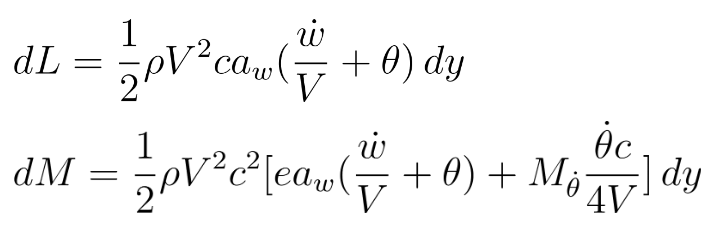with the lift coefficient function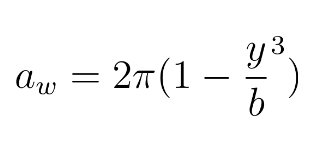where c is the local chord length (in x-direction) and b is the wingspan from the fixed root to tip (in y-direction). For the example we assume a constant load eccentricity factor “e” of 0.25, and a constant average “M_theta_dot” of -1.2.

We can then solve for our aerodynamic matrices by calculating the incremental work done by the aerodynamic forces over the surface of the wing and differentiating with respect to each of the generalised coordinates (i equations):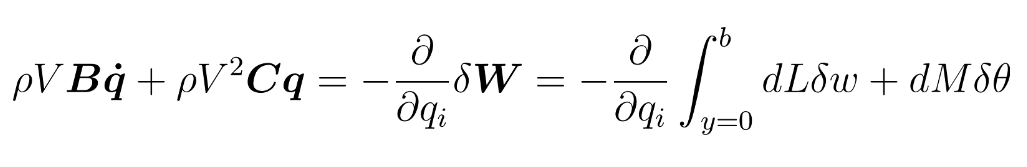where the incremental deflections and twist are sums over all k modes of the unknown coefficients “q” ( our generalised coordinates) multiplied by the eigenvectors for each mode: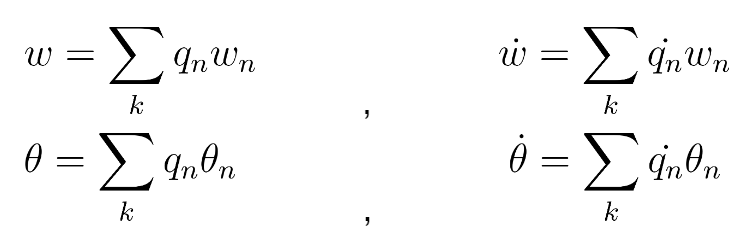The advantage of strip theory is that we can calculate the forces very quickly. However, it is a low fidelity model compared to CFD or even vortex lattice methods and it makes a lot of assumptions about the model which may not always be true. For example, we can show that the assumption of perfectly flat strips doesn’t hold for the higher frequency modes on our parametric wing model.

In the figure below we have plotted the first 4 mode shapes of the parametric wing with a [0/0/90]s layup. We can clearly see that there are some chordwise deformations happening on the structures model in mode 4 (and to a lesser degree in modes 2 and 3), which the aero model cannot capture as it only has 2 points defining each strip (one at the leading edge and one at the trailing edge).

Looking at these modes, we may decide that filling the wing with a foam core (at least partially) would probably be a good idea – and not only from a flutter point of view (think buckling!). In reality, most wings will have some sort of internal structure that will increase the panel vibration frequencies, but it is still something to keep in mind.

#### What is the effect of changing the composite material properties?

We looked at the effect of changing the main composite material fibre direction on the wing in some of our previous examples, showing that it affects the deflections and the twist of the wing under load and also the static aeroelastic loads on the wing.

So, what is the effect of the main fibre direction on the flutter and divergence velocities?

To answer this question, let’s look at the behaviour of the some wing model with the following composite laminates:

Reference design: [0 / 0 / 90]s
Washout design: [45 / 0 / 90]s
Washin design: [-45 / 0 / 90]s

The first 5 vibration frequencies and damping ratios for these designs are plotted in figure below. We can see that the reference design flutters at an airspeed of 70m/s, where the washout design flutters at 96m/s and the washin design flutters at 126m/s. However, the washin design also diverges at a lower airspeed of 121m/s, as the wing stiffness is not able to counteract the fast increasing aerodynamic forces on the outer wing.

In this case, the washin design seems to be best from an aeroelastic stability point of view. In general, it is more difficult to make any specific recommendations, but it is true that wings with washin tend to suffer from divergence type behaviours, whilst wings with washout tend to be flutter driven. Unfortunately, the only way to assess the actual behaviour is via simulation or testing…

#### In conclusion…

In this post we gave a brief introduction to aeroelastic flutter and divergence stability analysis, why it is important and how it can be implemented practically using a calculix finite element model coupled to a simplified dynamic strip theory aerodynamic model. We also investigated the effects of composite material induced elastic wing washout or washin on the aeroelastic stability. All our analyses assumed linear, small deflection behaviours.

As a next step, we might want to add a foam core to our model to stiffen the chordwise modes. We could also investigate using a higher fidelity aero model to verify some of our findings. We could also integrate the dynamic aeroelastic analysis into our design optimisation loop to ensure that our optimal wing design doesn’t flutter…To be continued!

#### From the author

Thanks for reading our blog. Feedback is a great way to learn, so if you have any questions or comments about this post or previous one, or if you are interested in a topic we haven’t covered yet, please get in touch! Olivia (Linkedin | Email)

Keywords

## Related PostsNews### Dapta partners with GStech

Announcement: Dapta partners with GStech to launch the Chinese version of the dapta cloud simulation platform.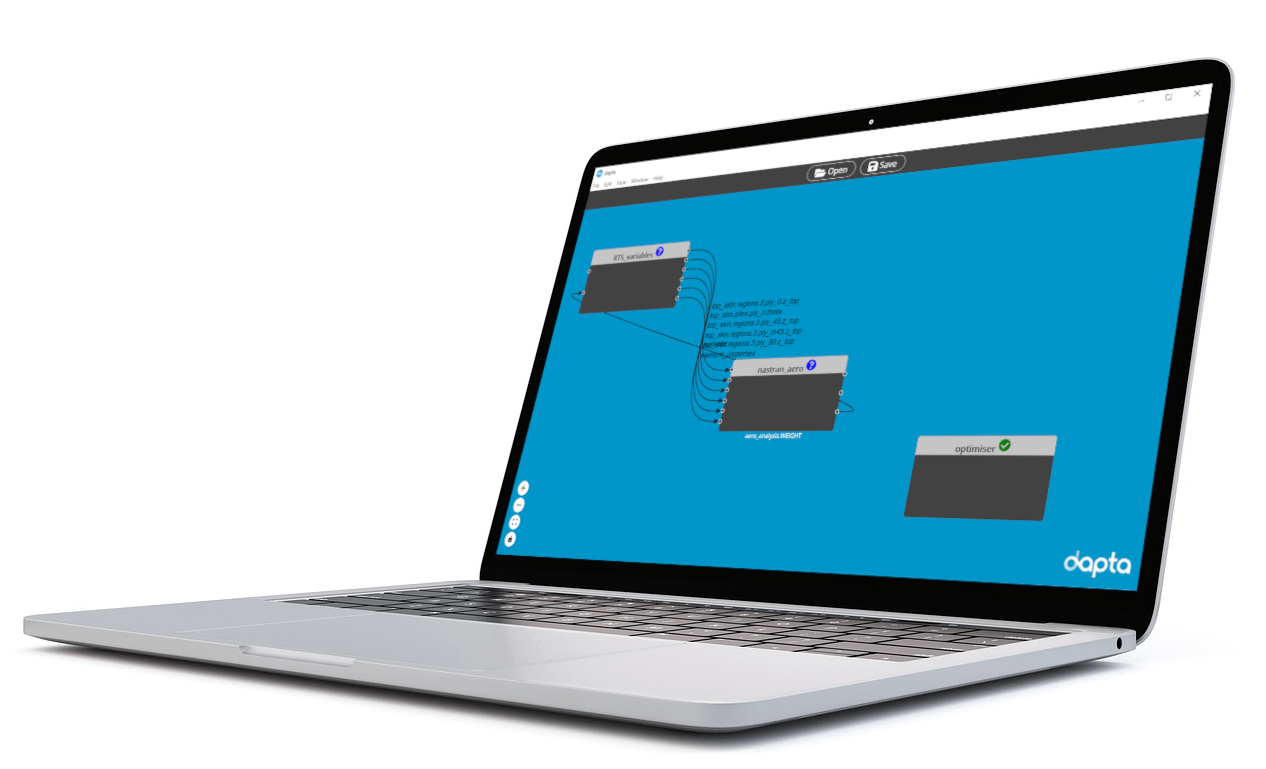News### Launching the Dapta Trial

Big News! After months of hard work, we can finally share a glimpse of what we have been working on. Not only that, but you can even try it out yourself. Here is an overview of what the Dapta app is all about.